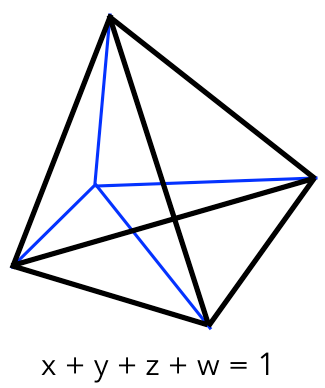# Math with my brother, part 6

Let’s review. We’re looking for a way to find a regular simplex — the simplest symmetric shape with flat boundaries — and we want our method to work no matter how many dimensions it has.

We already saw that to get a one dimensional simplex — a line — you go up to two dimensions and draw a line between the two points (1,0) and (0,1), keeping only the parts where all the coordinates are positive.

To get a two dimensional simplex — an equilateral triangle — you go up to three dimensions and draw a plane between the three points (1,0,0), (0,1,0) and (0,0,1), keeping only the parts where all the coordinates are positive.

It turns out this trick works in any number of dimensions. For example, suppose we want to get the three dimensional simplex — a regular tetrahedron.

You go up to four dimensions and draw a volume between the four points (1,0,0,0), (0,1,0,0), (0,0,1,0) and (0,0,0,1), keeping only the parts where all the coordinates are positive. In this picture, the x,y,z,w axes — all at right angles to each other — are in blue, and the resulting simplex is bounded by thick black lines:Wait, what was that? Draw a volume???

Well, sure. Math doesn’t care how many dimensions you have. It doesn’t even care whether you can picture in your head the thing you’re talking about. It just does exactly what you tell it to.

And now, thanks to the statistically inspired math my brother showed me, I have a way to create a regular simplex in any number of dimensions — even a hundred dimensions, or a million. It may not make any visual sense to me, but I can describe it exactly.

And when you think about it, that’s pretty cool.

Posted on